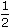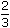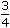7780831891 [email protected]

Instructions: This Monk test contains JK Bank Ratio and Proportion Problems with answer. This will help you in JK bank exam preparation.

This is a FREE online JK Bank Monk Test on topic JK Bank Ratio and Proportion Problems with answer. You DO NOT pay money to anyone to attend this test.
Each question carries 1 mark, no negative marks.
DO NOT refresh the page.
All the best :-).

Directions to Solve
In the following the questions choose the word which best expresses the meaning of the given word.

### #1. A and B together have Rs. 1210. If 4/15 of A's amount is equal to 2/5 of B's amount, how much amount does B have?

Explanation:

 4 A = 2 B 15 5A =2 x 15B 5 4A = 3 B 2A = 3 B 2A : B = 3 : 2.B’s share = Rs.1210 x 2= Rs. 484. 5

### #2. Two numbers are respectively 20% and 50% more than a third number. The ratio of the two numbers is:

Explanation:

Let the third number be x.

 Then, first number = 120% of x = 120x = 6x 100 5
 Second number = 150% of x = 150x = 3x 100 2Ratio of first two numbers =6x : 3x= 12x : 15x = 4 : 5. 5 2

### #3. A sum of money is to be distributed among A, B, C, D in the proportion of 5 : 2 : 4 : 3. If C gets Rs. 1000 more than D, what is B's share?

Explanation:

Let the shares of A, B, C and D be Rs. 5x, Rs. 2x, Rs. 4x and Rs. 3x respectively.

Then, 4x – 3x = 1000x = 1000.B’s share = Rs. 2x = Rs. (2 x 1000) = Rs. 2000.

### #4. Seats for Mathematics, Physics and Biology in a school are in the ratio 5 : 7 : 8. There is a proposal to increase these seats by 40%, 50% and 75% respectively. What will be the ratio of increased seats?

Explanation:

Originally, let the number of seats for Mathematics, Physics and Biology be 5x, 7x and 8x respectively.

Number of increased seats are (140% of 5x), (150% of 7x) and (175% of 8x).140 x 5x,150 x 7xand175 x 8x100 100 1007x, 21x and 14x. 2The required ratio = 7x : 21x : 14x 214x : 21x : 28x2 : 3 : 4.

### #5. In a mixture 60 litres, the ratio of milk and water 2 : 1. If this ratio is to be 1 : 2, then the quantity of water to be further added is:

Explanation:

 Quantity of milk =60 x 2litres = 40 litres. 3

Quantity of water in it = (60- 40) litres = 20 litres.

New ratio = 1 : 2

Let quantity of water to be added further be x litres.

 Then, milk : water =40. 20  x
 Now,40= 1 20  x 220  x = 80x = 60.Quantity of water to be added = 60 litres.

error decoding

### #6. The ratio of the number of boys and girls in a college is 7 : 8. If the percentage increase in the number of boys and girls be 20% and 10% respectively, what will be the new ratio?

Explanation:

Originally, let the number of boys and girls in the college be 7x and 8x respectively.

Their increased number is (120% of 7x) and (110% of 8x).120 x 7xand110 x 8x100 10042x and 44x 5 5The required ratio =42x : 44x= 21 : 22. 5 5

### #7. Salaries of Ravi and Sumit are in the ratio 2 : 3. If the salary of each is increased by Rs. 4000, the new ratio becomes 40 : 57. What is Sumit's salary?

Explanation:

Let the original salaries of Ravi and Sumit be Rs. 2x and Rs. 3x respectively.

 Then, 2x  4000 = 40 3x  4000 5757(2x  4000) = 40(3x  4000)6x = 68,0003x = 34,000

Sumit’s present salary = (3x  4000) = Rs.(34000 4000) = Rs. 38,000.

### #8. If 0.75 : x :: 5 : 8, then x is equal to:

Explanation:

 (x x 5) = (0.75 x 8)x =6= 1.20 5

### #9. The sum of three numbers is 98. If the ratio of the first to second is 2 :3 and that of the second to the third is 5 : 8, then the second number is:

Explanation:

Let the three parts be A, B, C. Then,

 A : B = 2 : 3 and B : C = 5 : 8 =5 x 3:8 x 3= 3 : 24 5 5 5A : B : C = 2 : 3 : 24 = 10 : 15 : 24 5B =98 x 15= 30. 49

### #10. If Rs. 782 be divided into three parts, proportional to 1/2 : 2/3 : 3/4, then the first part is:

Explanation:

Given ratio =::= 6 : 8 : 9.1st part = Rs.782 x 6= Rs. 204 23
error decoding

### #11. The salaries A, B, C are in the ratio 2 : 3 : 5. If the increments of 15%, 10% and 20% are allowed respectively in their salaries, then what will be new ratio of their salaries?

Explanation:

Let A = 2k, B = 3k and C = 5k.

 A’s new salary = 115 of 2k =115 x 2k= 23k 100 100 10
 B’s new salary = 110 of 3k =110 x 3k= 33k 100 100 10
 C’s new salary = 120 of 5k =120 x 5k= 6k 100 100New ratio23k : 33k : 6k= 23 : 33 : 60 10 10

### #12. If 40% of a number is equal to two-third of another number, what is the ratio of first number to the second number?

Explanation:

 Let 40% of A = 2 B 3
 Then, 40A = 2B 100 32A = 2B 5 3A =2 x 5= 5 B 3 2 3A : B = 5 : 3.

### #13. The fourth proportional to 5, 8, 15 is:

Explanation:

Let the fourth proportional to 5, 8, 15 be x.

Then, 5 : 8 : 15 : x5x = (8 x 15)

 x = (8 x 15) = 24. 5

### #14. Two number are in the ratio 3 : 5. If 9 is subtracted from each, the new numbers are in the ratio 12 : 23. The smaller number is:

Explanation:

Let the numbers be 3x and 5x.

 Then, 3x – 9 = 12 5x – 9 2323(3x – 9) = 12(5x – 9)9x = 99x = 11.The smaller number = (3 x 11) = 33.

### #15. In a bag, there are coins of 25 p, 10 p and 5 p in the ratio of 1 : 2 : 3. If there is Rs. 30 in all, how many 5 p coins are there?

 Then, sum of their values = Rs.25x 10 x 2x 5 x 3x= Rs. 60x 100 100 100 10060x = 30x = 30 x 100 = 50. 100 60